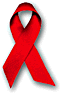World!Of
NumbersWON plate
154 |

December 1, 2003
December 1, 2003, not merely a date...
46664, not merely a palindrome...

December 1, 2003, not merely a date...
46664, not merely a palindrome...December 1st is worldAIDSday (link) www.46664.com is a vital campaign...

46664 features in this campaign not because it has a palindromic property
but because 46664 happened to be Nelson Mandela's prison number
on Robben Island where he was held captive for 18 years.
He gave his prison number to the campaign intended
to raise funds for Aids patients in South Africa.

A quote from his speech at the benefit concert [ Cape Town, Nov. 29, 2003 ]
"46664 is a vital campaign to help outfight a tragedy of
unprecedented proportions that is claiming more lives than
the sum total of all wars, famines and floods.
"Variation #11 ( from "ninedig3.htm" ) :
the equation  (A–B)^C + (D–E)^F + (G–H)^I

 46664 has 12 distinct solutions ! 46664 = (1–4)^2 + (3–9)^6 + (7–8)^5 46664 = (1–4)^2 + (7–8)^5 + (9–3)^6 46664 = (1–7)^6 + (3–4)^9 + (5–8)^2 46664 = (1–7)^6 + (3–4)^9 + (8–5)^2 46664 = (3–4)^9 + (5–8)^2 + (7–1)^6 46664 = (3–4)^9 + (7–1)^6 + (8–5)^2 46664 = (3–9)^6 + (4–1)^2 + (7–8)^5 46664 = (3–9)^6 + (5–7)^2 + (8–4)^1 46664 = (3–9)^6 + (7–5)^2 + (8–4)^1 46664 = (4–1)^2 + (7–8)^5 + (9–3)^6 46664 = (5–7)^2 + (8–4)^1 + (9–3)^6 46664 = (7–5)^2 + (8–4)^1 + (9–3)^6David Broadhurst (email) [November 27, 2004 ]
found a nice addendum to this 46664 topic
in the form of a very lovely palindromic prime
14666... ...66641 with 34111 digits.
Here is the full expression

(14665*1034110–56641)/9999

a probable prime for the time being.
it contains 8527 instances of Mandela's prison number 46664.
14666...66641 (34111-digits)A000154Prime Curios!Prime PuzzleWikipedia 154Le nombre 154```

```

[TOP OF PAGE]

Patrick De Geest - Belgium- Short Bio - Some Pictures